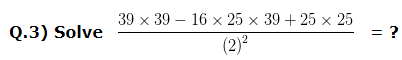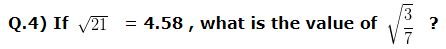# Squares and Square Roots Class 8 Worksheet

Download Squares and Square Roots Class 8 WorksheetQ.1) What is the least number by which 300 should be multiplied, so that the product of the two numbers would be a perfect square ?
a) 3
b) 9
c) 8

Q.2) What is the value of (92)²?
a) 8364
b) 8464
c) 8564a) 57
b) 49
c) 54a) 0.76
b) 0.65
c) 0.57

Q.5) If (26)² – (22)² = [(42)² – (40)²] x Y , then the value of Y is ?
a) 41/48
b) 48/41
c) 51/43

Q.6) Find the value of (56)² – (55)² + 4² ?
a) 147
b) 97
c) 127

Q.7) The value of (667)² – (666)² ?
a) 1333
b) 1343
c) 1353

Q.8) In a conference hall of a school, 225 students were sitting in such a way that there were as many rows as there were students in a row. How many rows were there?
a) 19
b) 15
c) 10

Q.9) A group of People decided to collect as many paisa from each member of the group as is the number of members. If the total collection amounts to Rs. 17.64 , the number of members in the group is ?
a) 45
b) 42
c) 38

Q.10) Which one of the following is perfect square ?
32402 , 38490 , 4900 , 31000
a) 32402
b) 38490
c) 4900

Q.1) a. 3
Q.2) b. 8464
Q.3) b. 49
Q.4) b. 0.65
Q.5) b. 48/41
Q.6) c. 127
Q.7) a. 1333
Q.8) b. 15
Q.9) b. 42
Q.10) c. 4900